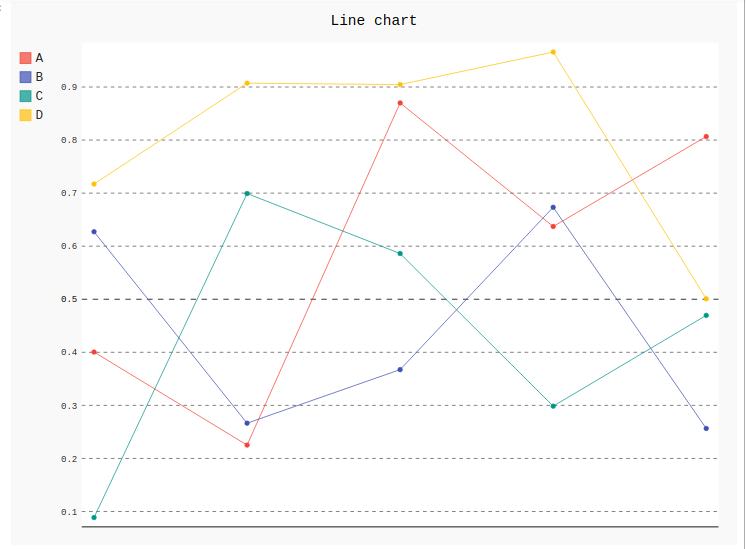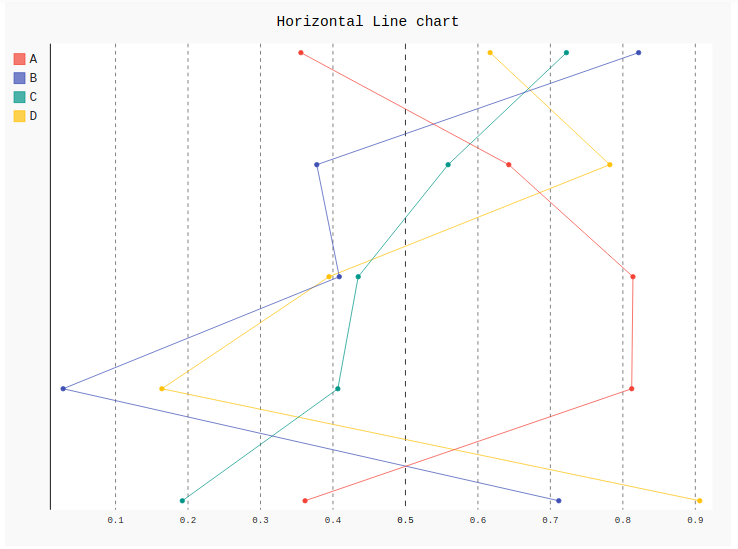# Line chart in Pygal

• Last Updated : 10 Jul, 2020

A line chart or line graph is a type of chart which helps to displays the information as a series of all data points called ‘markers’ and those markers are connected straight through line segments. It is a basic type of chart common in many fields which shows ascending or descending in a graph. A line chart is often used to visualize a trend in data over intervals of time which helps to represent the graph more effectively. Dimensions in the data are often displayed on the x-axis and y-axis.

## Creating Line Chart

1) Simple Line Chart: Simple basic line graphs are used for showing the relationship between two variables in vertical form. Line() method is used to create simple line graphs in Pygal.

Syntax:

`line_chart = pygal.Line()`

Example:

 `# importing pygal``import` `pygal``import` `numpy`` ` ` ` `# creating line chart object``line_chart ``=` `pygal.Line()`` ` `# naming the title``line_chart.title ``=` `'Line chart'`` ` ` ` `# adding lines``line_chart.add(``'A'``, numpy.random.rand(``5``))``line_chart.add(``'B'``, numpy.random.rand(``5``))``line_chart.add(``'C'``, numpy.random.rand(``5``))``line_chart.add(``'D'``, numpy.random.rand(``5``))`` ` `line_chart`

Output:2) Horizontal line chart: Horizontal line graph is the same as a basic line graph but the difference is it shows line horizontally from x-axis to y-axis. Both the variables are shown horizontally.

Syntax:

`line_chart = pygal.HorizontalLine()`

Example:

 `# importing pygal``import` `pygal``import` `numpy`` ` ` ` `# creating line chart object``line_chart ``=` `pygal.HorizontalLine()`` ` `# naming the title``line_chart.title ``=` `'Horizontal Line chart'`` ` ` ` `# adding lines``line_chart.add(``'A'``, numpy.random.rand(``5``))``line_chart.add(``'B'``, numpy.random.rand(``5``))``line_chart.add(``'C'``, numpy.random.rand(``5``))``line_chart.add(``'D'``, numpy.random.rand(``5``))`` ` `line_chart`

Output:My Personal Notes arrow_drop_up